Courses

# Important definitions and formulas - Areas Related to Circles Class 10 Notes | EduRev

## Class 10 : Important definitions and formulas - Areas Related to Circles Class 10 Notes | EduRev

The document Important definitions and formulas - Areas Related to Circles Class 10 Notes | EduRev is a part of the Class 10 Course Mathematics (Maths) Class 10.
All you need of Class 10 at this link: Class 10

⇒ Circumference of a circle or Perimeter of a circle:
The distance around the circle or the length of a circle is called its circumference or perimeter.
Circumference (perimeter) of a circle = πd or 2πr, where r is the radius of the circle and π=22/7 or 3.14.
⇒ Area of a circle:
Area of a circle = πr
⇒ Area of semicircle:
Area of semicircle =1/2 πr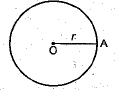Area of a quadrant:
Area of a quadrant (quarter circle) = πr2/4
⇒ Perimeter of semicircle:
Perimeter of a semicircle or protractor = πr+2r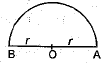Area of the ring:
Area of the ring or an annulus
= πR2 - πr2
= π(R2 - r2)
= π(R + r) (R - r)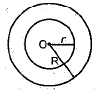⇒ Length of arc AB =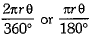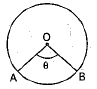⇒ Area of sector:
Area of sector OACBO =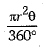or
Area of sector OACBO = 1/2(r x 1).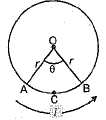⇒ Perimeter of sector:
Perimeter of sector OACBO
= length of arc AB + 2r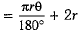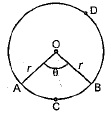⇒ Area of segment:
(i) Area of minor segment ACBA
= Area of sector OACBO - Area of ΔOAB =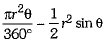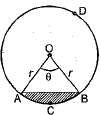(ii) Area of major segment BDAB
= Area of the circle - Area of minor segment ACBA
= πr2 - Area of minor segment ACBA.
⇒ If a chord subtend a right angle at the centre, then
Area of the corresponding segment =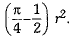⇒ If a chord subtend an angle of 60° at the centre, then
Area of the corresponding segment =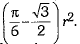⇒ If a chord subtend an angle of 120° at the centre, then
Area of the corresponding segment =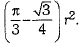⇒ Other important formulae:
(i) Distance moved by a wheel in 1 revolution = Circumference of the wheel
(ii) Number of revolutions in one minute =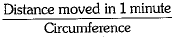(iii) Angle described by the minute hand in 60 minutes = 360°
(iv) Angle described by the hour hand in 12 hours = 360°
⇒ The mid-point of the hypotenuse of a right triangle is equidistant from the vertices of the triangle.
⇒ Angle subtended at the circumference by a diameter is always a right angle.

Offer running on EduRev: Apply code STAYHOME200 to get INR 200 off on our premium plan EduRev Infinity!

## Mathematics (Maths) Class 10

62 videos|363 docs|103 tests

,

,

,

,

,

,

,

,

,

,

,

,

,

,

,

,

,

,

,

,

,

;